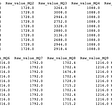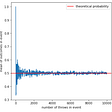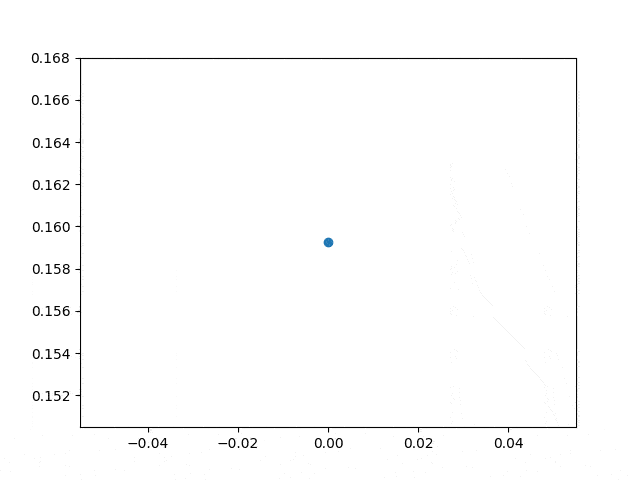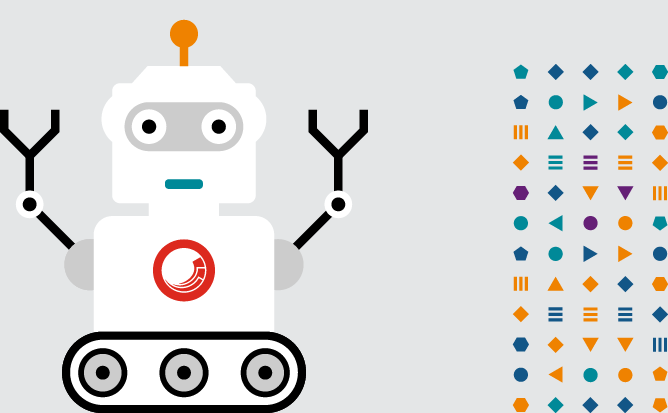# KMeans：能從資料中找出 K 個分類的非監督式機器學習演算法 — — 所以它到底有啥用？（使用 scikit-learn 與 Python）

`import matplotlib.pyplot as pltfrom sklearn.datasets import make_blobsfrom sklearn.cluster import KMeans# 隨機產生 10 組 2 features 的資料 500 筆 (dy 即原始目標值 label 0~9)dx, dy = make_blobs(n_samples=500, n_features=2, centers=10, random_state=42)# 用 KMeans 在資料中找出 5 個分組kmeans = KMeans(n_clusters=5)kmeans.fit(dx)# 預測新的目標值 labelnew_dy = kmeans.predict(dx)plt.rcParams['font.size'] = 14plt.figure(figsize=(16, 8))# 以不同顏色畫出原始的 10 群資料plt.subplot(121)plt.title('Original data (10 groups)')plt.scatter(dx.T, dx.T, c=dy, cmap=plt.cm.Set1)# 根據重新分成的 5 組來畫出資料plt.subplot(122)plt.title('KMeans=5 groups')plt.scatter(dx.T, dx.T, c=new_dy, cmap=plt.cm.Set1)# 顯示圖表plt.tight_layout()plt.show()`

`import matplotlib.pyplot as pltfrom mpl_toolkits.mplot3d import Axes3D # matplotlib 3.2.0 後可省略from sklearn.datasets import make_blobsfrom sklearn.cluster import KMeansdx, dy = make_blobs(n_samples=500, n_features=3, centers=10, random_state=42)kmeans = KMeans(n_clusters=5)kmeans.fit(dx)new_dy = kmeans.predict(dx)plt.rcParams['font.size'] = 14fig = plt.figure(figsize=(16, 8))ax = fig.add_subplot(121, projection='3d')plt.title('Original data (10 groups)')ax.scatter(dx.T, dx.T, dx.T, c=dy, cmap=plt.cm.Set1)ax = fig.add_subplot(122, projection='3d')plt.title('KMeans=5 groups')ax.scatter(dx.T, dx.T, dx.T, c=new_dy, cmap=plt.cm.Set1)plt.show()`

`import matplotlib.pyplot as pltfrom sklearn.datasets import make_blobsfrom sklearn.metrics import silhouette_scorefrom sklearn.cluster import KMeanscolors = ['red', 'orange', 'yellow', 'green', 'cyan',          'blue', 'purple', 'brown', 'grey', 'black']# 產生的資料組數 (10)clusters = 10# K 值的範圍 (2~10)k_range = range(2, clusters + 1)dx, dy = make_blobs(n_samples=500, n_features=2, centers=clusters, random_state=42)distortions = []scores = []# 記錄每種 K 值建出的 KMeans 模型的成效for i in k_range:    kmeans = KMeans(n_clusters=i).fit(dx)    distortions.append(kmeans.inertia_) # 誤差平方和 (SSE)    scores.append(silhouette_score(dx, kmeans.predict(dx))) # 側影係數# 找出最大的側影係數來決定 K 值selected_K = scores.index(max(scores)) + 2# 重新建立 KMeans 模型並預測目標值kmeans = KMeans(n_clusters=selected_K).fit(dx)new_dy = kmeans.predict(dx)# 新分組的資料中心點centers = kmeans.cluster_centers_plt.rcParams['font.size'] = 12plt.figure(figsize=(12, 12))# 原始資料分組plt.subplot(221)plt.title(f'Original data ({clusters} groups)')plt.scatter(dx.T, dx.T, c=dy, cmap=plt.cm.Set1)# 新資料分組plt.subplot(222)plt.title(f'KMeans={selected_K} groups')plt.scatter(dx.T, dx.T, c=new_dy, cmap=plt.cm.Set3)plt.scatter(centers.T, centers.T, marker='^', color='orange')for i in range(centers.shape): # 標上各分組中心點    plt.text(centers.T[i], centers.T[i], str(i + 1),             fontdict={'color': 'red', 'weight': 'bold', 'size': 24})# 繪製誤差平方和圖 (手肘法)plt.subplot(223)plt.title('SSE (elbow method)')plt.plot(k_range, distortions)plt.plot(selected_K, distortions[selected_K - 2], 'go') # 最佳解# 繪製係數圖plt.subplot(224)plt.title('Silhouette score')plt.plot(k_range, scores)plt.plot(selected_K, scores[selected_K - 2], 'go') # 最佳解plt.tight_layout()plt.show()`

`import matplotlib.pyplot as pltimport seaborn as snsimport pandas as pdfrom sklearn import datasetsfrom sklearn.metrics import silhouette_scorefrom sklearn.cluster import KMeansdata = datasets.load_boston()# 把波士頓房價的 data 和 target 合併成一個 pandas DataFramedx = pd.DataFrame(data['data'], columns=data['feature_names'])dy = pd.DataFrame(data['target'], columns=['MEDV'])df = pd.concat((dy, dx), axis=1)# 最大 K 值K_max = 20# 計算側影係數scores = []for i in range(2, K_max + 1):    scores.append(        silhouette_score(            df, KMeans(n_clusters=i).fit_predict(df)))# 得出最佳 K 值selected_K = scores.index(max(scores)) + 2print('K =', selected_K, '\n')# 對房價分組kmeans = KMeans(n_clusters=selected_K)labels = kmeans.fit_predict(df)# 將分組資料 (分類標籤) 併入原資料lb = pd.DataFrame(labels, columns=['labels'])df = pd.concat((lb, df), axis=1)# 印出標籤和房價、以及其統計結果print(df[['labels', 'MEDV']], '\n')print('原始資料\n', df['MEDV'].describe(), '\n')# 抽出不同組的房價並印出統計結果df_group = []for i in range(selected_K):    df_new = df[df['labels']==i]['MEDV']    print(f'分類 {i + 1}\n', df_new.describe(), '\n')    df_group.append(df_new)# 用 seaborn 畫出所有組別房價的箱型圖sns.boxplot(data=df_group)plt.show()`

`K = 3labels  MEDV0         1  24.01         1  21.62         1  34.73         1  33.44         1  36.2..      ...   ...501       1  22.4502       1  20.6503       1  23.9504       1  22.0505       1  11.9[506 rows x 2 columns]原始資料 count    506.000000mean      22.532806std        9.197104min        5.00000025%       17.02500050%       21.20000075%       25.000000max       50.000000Name: MEDV, dtype: float64分類 1 count    366.000000mean      24.931694std        8.334857min       11.80000025%       19.50000050%       22.80000075%       28.575000max       50.000000Name: MEDV, dtype: float64分類 2 count    102.000000mean      17.429412std        9.184539min        5.00000025%       12.15000050%       15.30000075%       20.600000max       50.000000Name: MEDV, dtype: float64分類 3 count    38.000000mean     13.126316std       4.395310min       7.00000025%       9.75000050%      13.25000075%      14.975000max      27.500000Name: MEDV, dtype: float64`

`import numpy as npimport matplotlib.pyplot as plt # 需安裝 pillow 才能讀 JPEGfrom matplotlib import imagefrom sklearn.cluster import MiniBatchKMeans# K 值 (要保留的顏色數量)K = 64# 讀取圖片image = image.imread(r'C:\Users\使用者名稱\Downloads\photo.jpg') / 255w, h, d = tuple(image.shape)image_data = np.reshape(image, (w * h, d))# 將顏色分類為 K 種kmeans = MiniBatchKMeans(n_clusters=K, batch_size=5000)labels = kmeans.fit_predict(image_data)centers = kmeans.cluster_centers_# 根據分類將顏色寫入新的影像陣列image_compressed = np.zeros(image.shape)label_idx = 0for i in range(w):    for j in range(h):        image_compressed[i][j] = centers[labels[label_idx]]        label_idx += 1# 如果想儲存壓縮後的圖片, 將下面這句註解拿掉#plt.imsave(r'C:\Users\使用者名稱\Downloads\compressed.jpg', image_compressed)# 顯示原圖跟壓縮圖的對照plt.figure(figsize=(12, 9))plt.subplot(121)plt.title('Original photo')plt.imshow(image)plt.subplot(122)plt.title(f'Compressed to KMeans={K} colors')plt.imshow(image_compressed)plt.tight_layout()plt.show()`

• 原圖：1.83 MB
• K=64：390 KB
• K=16：379 KB
• K=4：330 KB

--

--

--

## More from Alan Wang

My writing power is 100% generated by free coffee. (github.com/alankrantas, www.hackster.io/alankrantas)

Love podcasts or audiobooks? Learn on the go with our new app.## Alan Wang

My writing power is 100% generated by free coffee. (github.com/alankrantas, www.hackster.io/alankrantas)

## Processing of MQ-X sensors’ data with Machine Learning.## The Beta Distribution explained through Binomial and Bernoulli Distribution## Dynamic Graph Plotting — Matplotlib## Cluster Analysis-Unsupervised ML for Pairs Trading using Indian Stock data